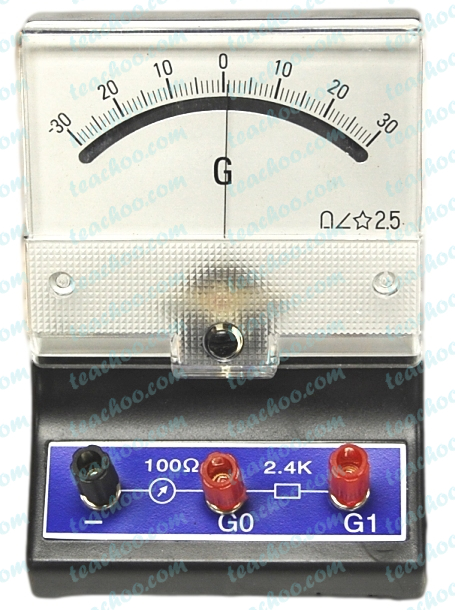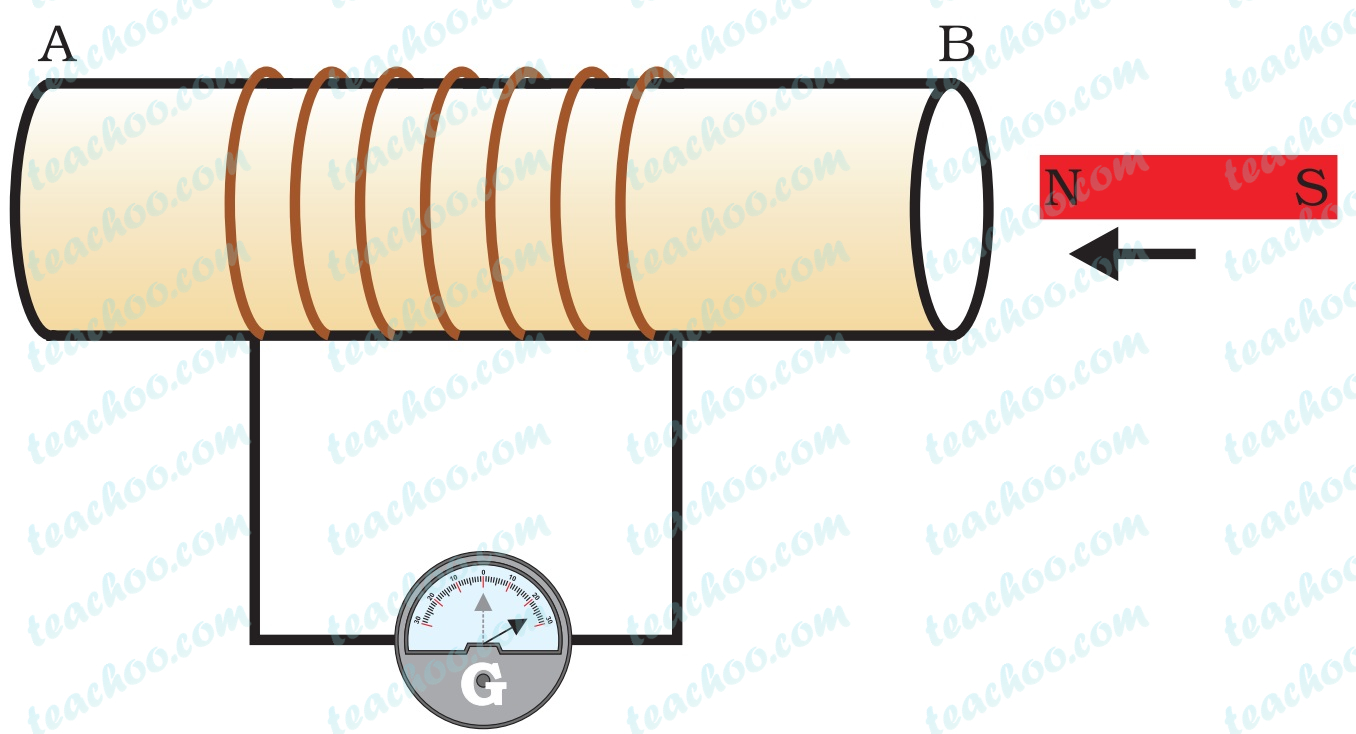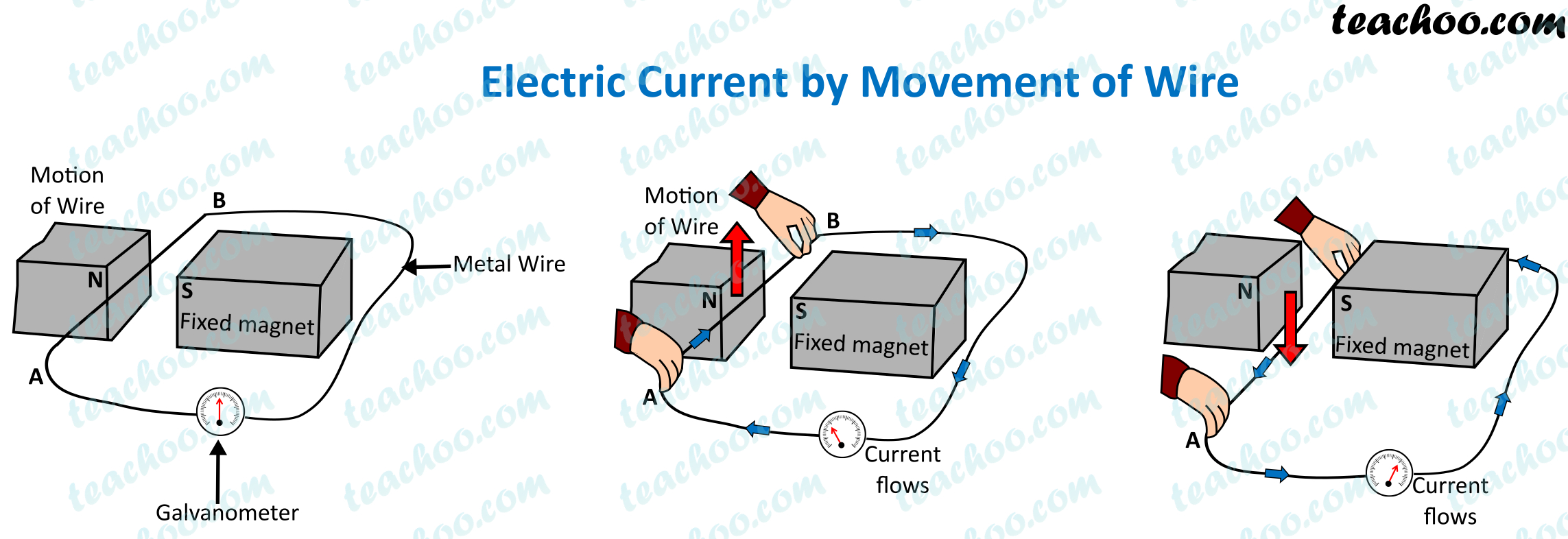Concepts

Class 10
Chapter 13 Class 10 - Magnetic Effects of Electric Current

Current carrying Wire Causes Magnet to Move

Current Carrying Wire causes Magnetic Compass to Deflect

Hence, Current Carrying Wire creates magnetic field around it

Later we studied
If Current Carrying Wire is kept in a Magnetic Field,
it experiences a force

Now we are learning that

We can produce current by

• Moving conducting Wire inside a Fixed Magnetic Field
or
• Keeping Conductor Fixed and Changing Magnetic Field

This is called ElectroMagnetic Induction

## What is Electromagnetic Induction?

The production of Electricity from Magnetism is called Electromagnetic Induction

We can produce current by

• Moving conducting Wire inside a Fixed Magnetic Field
(Hence, if we keep a conducting wire near the magnetic field of magnet and move it vigorously, current will be induced in it.)
or
• Keeping Wire (Conductor) Fixed and Changing Magnetic Field
(Hence, if keep a wire fixed and change poles of magnet vigorously, current will be induced in wire)

This is called ElectroMagnetic Induction

## Who discovered ElectroMagnetic Induction?

It was discovered by Michael Faraday

This discovery helped in generation of electric generators which supply electricity to our homes

## What is Induced Current?

Current which is produced by Electromagnetic Induction is called Induced Current

Hence, this type of current is produced by either movement of wire or change in magnetic field

Induced current is highest when direction of motion of coil is at right angle to the direction of magnetic field.

Explanation

The word induced means produced without any direct contact with physical body

Induced current means current produced by movement of wire (conductor) in a magnetic field or change in magnetic field (This current is produced without any physical contact between wire and magnet)

## How to Find Direction of Induced Current?

Direction of induced current is found with the help of Fleming's right hand rule

## How is current measured in a circuit?

It is measured with the help of a device called galvanometer

### What is a Galvanometer?Galvanometer is a device which detects presence of current in circuit

If no current flows in circuit, pointer of galvanometer remains at 0

If current flows in circuit, it deflects either left or right (depending upon flow of current in circuit)

It is denoted by G in a circuit.

## Activity to Show Electromagnetic Induction with the help of Galvanometer• We take a coil of wire (having large number of turns)

• We connect it to a galvanometer
Note - There is no battery attached to the circuit

• We bring strong magnet near the wire

• Slowly we start moving it towards one side of wire
We see deflection in galvanometer
This is because movement of magnet has created an electric current

• Now we stop moving the magnet
We notice that deflection of galvanometer also stops
This shows that when movement of magnet stops, generation of current also stops

• Now we start moving magnet in opposite direction
We notice that galvanometer again deflects but in opposite direction
This shows that direction of movement of magnet effects direction of movement of electric current

Here's a GIF of the experiment## Activity to Induce Current by Movement of Wire• We make a circuit by attaching wire to the Galvanometer

• Now we take a horse shoe magnet
and put the wire between the poles of the horse shoe magnet

• Currently the wire is not moving
Note that currently there is no deflection in galvanometer

• But if we move wire upwards,
we see deflection in galvanometer

• And if we move wire downwards,
we again see deflection in galvanometer but in the opposite side

So, current is induced by changing movement of wire

## Which above 2 methods is generally used to generate current?

Generating Current by Movement of Wire

or

Generating current by Changing Magnetic Field

Generating Current by Movement of Wire is generally used

## Questions

NCERT Question 2 - The phenomenon of electromagnetic induction is

1. the process of charging a body.
2. the process of generating magnetic field due to a current passing through a coil.
3. producing induced current in a coil due to relative motion between a magnet and the coil.
4. the process of rotating a coil of an electric motor

NCERT Question 13 - A coil of insulated copper wire is connected to a galvanometer. What will happen if a bar magnet is (i) pushed into the coil, (ii) withdrawn from inside the coil, (iii) held stationary inside the coil?

NCERT Question 14 - Two circular coils A and B are placed close to each other. If the current in the coil A is changed, will some current be induced in the coil B? Give reason.

NCERT Question 15 (iii) - State the rule to determine the direction of a

current induced in a coil due to its rotation in a magnetic field.

Q1 Page 236 - Explain different ways to induce a current in a coil.

Learn in your speed, with individual attention - Teachoo Maths 1-on-1 Class